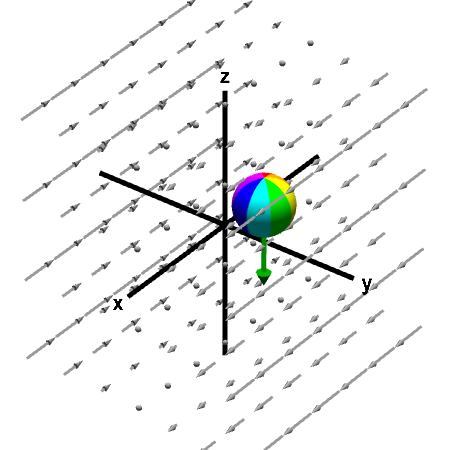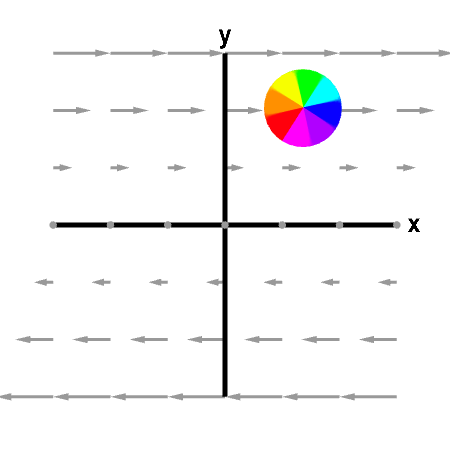# Math Insight

### Applet: Sphere rotating in shear flowWhen the center of the sphere is fixed, it rotates in a clockwise direction when viewed from the positive $z$-axis, corresponding to a downward pointing curl (green arrow). On the other hand, the vector field is near the sphere is pointing straight in positive $x$ direction. Although the vector field does not appear to be rotating, the change in velocity as one moves perpendicular to the flow still leads to the microscopic circulation captured by curl. First panel shows the full vector field; second panel shows its projection in the $xy$-plane.

This vector field is $\dlvf(x,y,z) = (y,0,0)$. One can compute that $\curl \dlvf(x,y,z) = (0,0,-1)$.

You can move the sphere by dragging it with the mouse. Since for this particular vector field, the curl does not change with position, the rotation of the sphere is unchanged as you move it around.